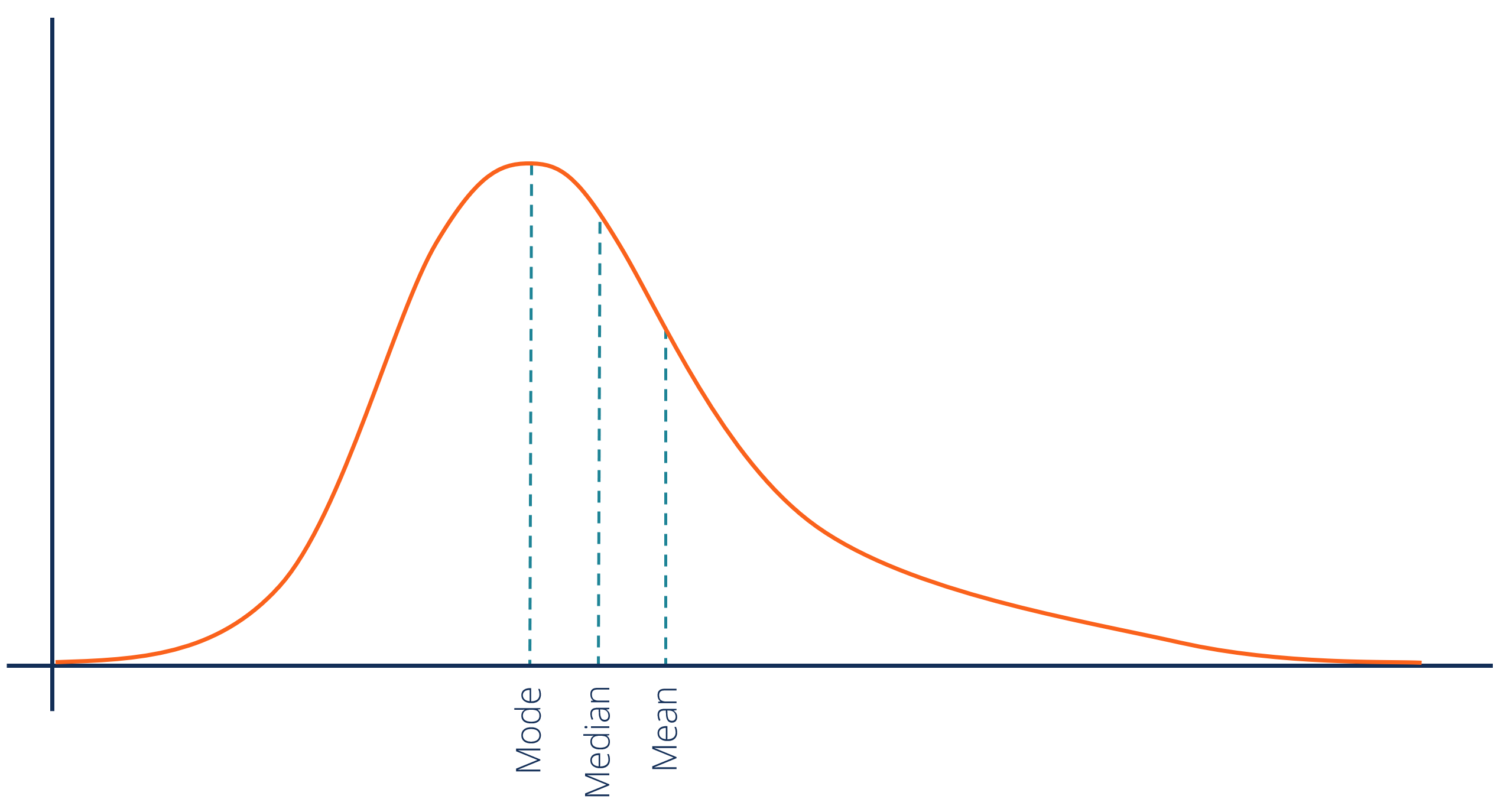# Positively Skewed Distribution

A type of distribution in which most values are clustered around the left tail of the distribution

## What is a Positively Skewed Distribution?

In statistics, a positively skewed (or right-skewed) distribution is a type of distribution in which most values are clustered around the left tail of the distribution while the right tail of the distribution is longer. The positively skewed distribution is a direct opposite of the negatively skewed distribution.### Central Tendency Measures in Positively Skewed Distributions

Unlike the normally distributed data where all measures of the central tendency (mean, median, and mode) equal each other, in a positively skewed data, the measures are dispersed. The general relationship among the central tendency measures in the positively skewed distribution may be expressed using the following inequality:

##### Mean  >  Median  >  Mode

In contrast with a negatively skewed distribution, in which the mean is located on the left from the peak of distribution, in a positively skewed distribution, the mean can be found on the right from the distribution’s peak. However, not all negatively skewed distributions follow the rules. You may encounter many exceptions in real life that violate the rules.

Since a high level of the skewness can generate misleading results from statistical tests, the extreme positive skewness is not desirable for a distribution. In order to overcome such a problem, data transformation tools may be employed to make the skewed data closer to the normal distribution.

For positively skewed distributions, the most popular transformation is the log transformation. The log transformation implies the calculations of the natural logarithm for each value in the dataset. The method allows reducing the skew of a distribution. Statistical tests are usually run only when the transformation of the data is complete.

### Positively Skewed Distribution in Finance

In finance, the concept of skewness is utilized in the analysis of the distribution of the returns of investments. Although many finance theories and models assume that the returns from securities follow the normal distribution, in reality, the returns are usually skewed.

The positive skewness of a distribution indicates that an investor may expect frequent small losses and few large gains from the investment. The positively skewed distributions of investment returns are generally more desirable by investors since there is some probability to gain huge profits that can cover all the frequent small losses.